诡秘迷宫

正文第一章 法律培训班

[更新时间] 2018-11-29 05:40:01 [字数] 1028

“同学们，司法考试，又称天下第一考，现在正式改名为法律职业资格考试……”+#^=\$想要阅!读更^多|精*彩^内容\$，请^登#录\$纵*横中^文~网www.zongheng.com|*\$+^

“李，李老，师！”+#^=\$想要阅!读更^多|精*彩^内容\$，请^登#录\$纵*横中^文~网www.zongheng.com|*\$+^

“史悦，你放弃吧，不适合做法官，检察官！”+#^=\$想要阅!读更^多|精*彩^内容\$，请^登#录\$纵*横中^文~网www.zongheng.com|*\$+^

“律，律师？”+#^=\$想要阅!读更^多|精*彩^内容\$，请^登#录\$纵*横中^文~网www.zongheng.com|*\$+^

“律师更不适合！总之你适合我们法律工作者！”+#^=\$想要阅!读更^多|精*彩^内容\$，请^登#录\$纵*横中^文~网www.zongheng.com|*\$+^

“救命啊！”+#^=\$想要阅!读更^多|精*彩^内容\$，请^登#录\$纵*横中^文~网www.zongheng.com|*\$+^

“你来了！”+#^=\$想要阅!读更^多|精*彩^内容\$，请^登#录\$纵*横中^文~网www.zongheng.com|*\$+^

“同学们，今天的课程先讲到这儿，明天我会把今天的内容，给大家补上！”+#^=\$想要阅!读更^多|精*彩^内容\$，请^登#录\$纵*横中^文~网www.zongheng.com|*\$+^

“傻子，你还不走？要不是这个培训班通过率高，谁会在这儿学，我现在就是希望可以快点考完！我好回家！”+#^=\$想要阅!读更^多|精*彩^内容\$，请^登#录\$纵*横中^文~网www.zongheng.com|*\$+^

“别看了，傻子，快走吧！我们今天中午吃什么？对了！给你一个惊喜！”+#^=\$想要阅!读更^多|精*彩^内容\$，请^登#录\$纵*横中^文~网www.zongheng.com|*\$+^

“同学，你俩要报班嘛？想学什么？市面上卖的全部课程，我都有，可是只卖2980元，知识可以带回家，你们看看……”+#^=\$想要阅!读更^多|精*彩^内容\$，请^登#录\$纵*横中^文~网www.zongheng.com|*\$+^

“躲开！”+#^=\$想要阅!读更^多|精*彩^内容\$，请^登#录\$纵*横中^文~网www.zongheng.com|*\$+^

“哇！漂，漂亮！”+#^=\$想要阅!读更^多|精*彩^内容\$，请^登#录\$纵*横中^文~网www.zongheng.com|*\$+^

“傻子，我答应你，等你过生日时，带你来一个地方，没骗你吧？”+#^=\$想要阅!读更^多|精*彩^内容\$，请^登#录\$纵*横中^文~网www.zongheng.com|*\$+^

“史悦，快回来，这样很危险！过来看我为你准备了什么？”+#^=\$想要阅!读更^多|精*彩^内容\$，请^登#录\$纵*横中^文~网www.zongheng.com|*\$+^

“史悦，生日快乐！”+#^=\$想要阅!读更^多|精*彩^内容\$，请^登#录\$纵*横中^文~网www.zongheng.com|*\$+^

“谢，谢谢，谢！”+#^=\$想要阅!读更^多|精*彩^内容\$，请^登#录\$纵*横中^文~网www.zongheng.com|*\$+^

“好了，傻子，不用说这么多声谢谢，其实，我有时发现你这口吃还挺好的，至少在这个时候！”+#^=\$想要阅!读更^多|精*彩^内容\$，请^登#录\$纵*横中^文~网www.zongheng.com|*\$+^

“美，美美，美琳！”+#^=\$想要阅!读更^多|精*彩^内容\$，请^登#录\$纵*横中^文~网www.zongheng.com|*\$+^

“对了！还有叫我名字的时候，哈哈哈，傻子，你怎么哭了，别告诉我你是第一次过生日？”+#^=\$想要阅!读更^多|精*彩^内容\$，请^登#录\$纵*横中^文~网www.zongheng.com|*\$+^

[+展开]

发表评论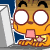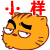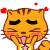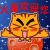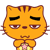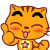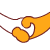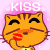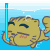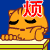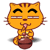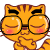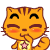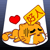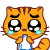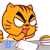12